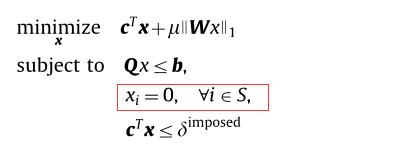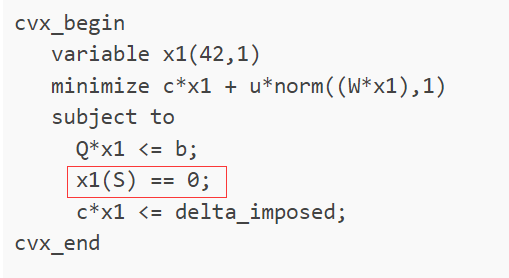How can I write this expression?where S is a set

It depends how you will represent S in your code. Standard Matlab indexing applies. So if say `S=[1 3 6]` then you can write `x(S)==0`.

I need to iterate through this cvx program, but writing this constraint like this doesn’t seem to work

Please share a reproducible code sample that doesn’t work if you want help with cvx syntax.

``````N=80;
wpass = 0.0436;
wstop = 0.0872;
dpass = 0.06;
dstop = 0.1;
w = linspace(0,pi,300);
% create optimization matrices (this is cosine matrix)
C = [ones(300,1) 2*cos(kron(w',[1:N/2]))];
Hdes = [ones(1,sum(w<=wpass*pi)),zeros(1,sum(w>wpass*pi))]';

maxsteps = 15;
alpha  = 1e-6;
alpha_stop = 1e-4;
alpha_hard = 1e-7;
u =0.1;

global x
global x1
v = ones(300,1);
c = [(zeros(41,1));1]';
b = [Hdes;-Hdes];
Q = [C,-v;-C,-v];
on = [ones(1,41),0];
W = diag(on);

cvx_begin
variable x(42,1)
minimize c*x
subject to
Q*x <= b;
cvx_end
delta = x(42);
delta_imposed = 2*delta;

for K = 1:maxsteps

cvx_begin
variable x1(42,1)
minimize c*x1 + u*norm((W*x1),1)
subject to
Q*x1 <= b;
x1(S) == 0;
c*x1 <= delta_imposed;
cvx_end

x1_old = zeros(42,1);
relerr = sqrt(sum((x1-x1_old).^2));
x1_old = x1;

for  i = 1:41
for  j = 1:41
if(i==j)
W(i,j) = 1/abs(x1(i)+eps);
else
W(i,j) = 0;
end
end
end
S = find(abs(x1)<=alpha_hard);

end

``````

Thank you!

And what is the problem/error that you encounter with this code?The red code is not executed as a constraint

``````x1
S
x1(S)
``````

in every loop of your iteration and I see that `x1(S)` is zero all the time. So to me the constraint works.

You only show code to set `S` after the CVX optimization problem in which it is used. Are you not showing all the code? Or perhaps you forgot to set an initial value of `S` before you used it in the CVX constraint? Given your CVX code, `S` needs to be an array of integers, each in the range of 1 to 42.

The first help you need is to understand the problem you found in a paper or book before you attempt to implement it in CVX… That is for you to do, perhaps with help from your advisor or the model author(s); not something for CVX forum readers to do.

I displayed all the code, but I didn’t know it was not quite correct when I executed it myself.

If you never set `S` before using it foe rhe first time, how can you expect the code to be correct?

Thank you for your reply. I will carefully check my code. Thank you sincerely!